# Mechanical Engineering - Heat Transfer, Refrigeration and Air Conditioning

### Exercise :: Heat Transfer, Refrigeration and Air Conditioning - Section 1

11.

According to Dalton's law of partial pressures, (where pb = Barometric pressure, pa = Partial pressure of dry air, and pv = Partial pressure of water vapour)

 A. Pb = pa - pv B. Pb = pa + pv C. Pb = pa x pv D. Pb = pa/pv

Explanation:

No answer description available for this question. Let us discuss.

12.

The heat transfer by conduction through a thick sphere is given by

 A.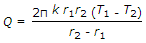B.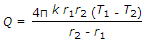C.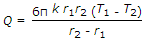D.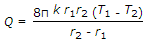Explanation:

No answer description available for this question. Let us discuss.

13.

The relative humidity is defined as

 A. the mass of water vapour present in 1 m3 of dry air B. the mass of water vapour present in 1 kg of dry air C. the ratio of the actual mass of water vapour in a unit mass of dry air to the mass of water vapour in the same mass of dry air when it is saturated at the same temperature and pressure. D. the ratio of actual mass of water vapour in a given volume of moist air to the mass of water vapour in the same volume of saturated air at the same temperature and pressure

Explanation:

No answer description available for this question. Let us discuss.

14.

Fourier's law of heat conduction is (where Q = Amount of heat flow through the body in unit time, A = Surface area of heat flow, taken at right angles to the direction of heat flow, dT = Temperature difference on the two faces of the body, dx = Thickness of the body, through which the heat flows, taken along the direction of heat flow, and k = Thermal conductivity of the body)

 A.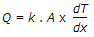B.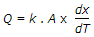C.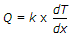D.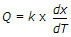Explanation:

No answer description available for this question. Let us discuss.

15.

The heat transfer by conduction through a thick sphere is same as through a thick cylinder.

 A. True B. False